# How to Make Circular and Polygon Radial Charts

I recently got inspired from viewing a dashboard designed by Adam Crahen, where he created a Circular Radial Chart based on data about Gender Disparity in Australia *. Doing some research I came up with a few posts that explain how to create these types of charts, such as here and here.

In this post, my goal is to expand this type of chart from circular to a polygon shape using Tableau. We will use the line drawing capabilities to create each of the bars.

A Polygon Radial Chart is useful for visualizing distinct groups of data.

A. DATA

The data used is located here. Our data consists of columns with the Occupation, Gender, and the Average Taxable Income \$. Most occupations appear twice, since it presents the average income obtained by Males and Females. For example, Actors make an average of \$34,736 and \$36,624 for Females and Males, respectively; which represents a Gap of \$1,888.In the radial chart, each bar represents an Occupation, and the length of the bar is proportional to the salary Gap between Males and Females.

There are several steps in common for creating a Circular and Polygon Radial Chart. First, we need to understand the image below, where we describe the Radius of the circle, the Angle where each bar will be drawn, the length of the bar which is described as the normalized gap (NormGap), and finally the X and Y coordinates for the start and end points of the bar (XCircle and YCircle). To create this chart, we need to know the values of XCircle and YCircle. In the following sections, we will describe how to obtain these coordinates using the other measures (e.g. Radius, NormGap, etc.).B.1. BAR LENGTH

The length of the bar should be proportional to the Gap between Male and Female salaries per Occupation.To get this into Tableau we will create two Calculated Fields (CF) which separate the Male and Female Data (MaleData and FemaleData). Then, we use those values to calculate the Gap. Note that positive values indicate occupations where Males make more money, and negative values indicate the opposite.

The Gap has units in \$. However, we want to rescale the data so that the Gap will have a value between -1 and 1. To normalize the data we divide the Gap by its largest absolute value.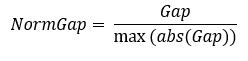B.2. FILTER

The table below shows the results of the CFs previously obtained. When we look at the data, we notice some occupations with Null values in the NormGap. This occurs when we have occupations where only one gender appears. In the example below, only Females have taken on the role of Mothercraft nurse. Since we will not be using this data, we will include the Gap field in the Filter and remove all the Null values.B.3. INDEX

We need a CF that contains an index for each occupation (IndexCalc). The Index() function will assign a unique value to each row, not to each occupation. Therefore, our calculation consists of dividing the Index by 2 if the value is even, and dividing by 2 and adding 1 if the value is odd.B.4. PATH ORDER

Since we are using Tableau’s line drawing tool to create each bar, it is required to indicate a path order for the line. We will assign 0 and 1 to the start and end points, respectively. If the Gap is positive, we will assign the PathOrder of 1 to Male and 0 to Female. The opposite will occur if the Gap is negative.We define a parameter Radius which contains the radius of the circle. Each bar will be initiated at a point around the perimeter of this circle.B.6. ANGLE OF EACH BAR

We will assign each occupation to a position on the perimeter of the circle made with Radius. Each occupation will be assigned an angle between 0 and 2*Pi. This is calculated using:C.1. X and Y COORDINATES

We now determine the X and Y coordinates for the start and end points of each bar. As a reminder, values with a PathOrder of 0 and 1 represent the start and end points, respectively. Since we know the Angle and Radius for each occupation on the circle, we use trigonometry to perform the calculation of the start and end points. For the end points we extended the Radius by adding the NormGap. The equations are given by:The following section is specific to the Polygon Radial Chart. In the image below we present a pentagon, which has 5 sides. The same analysis applies for other number of sides. The vertices of each side are located at a distance of Radius. Each side of the pentagon has 2 vertices, located at angles of AngleStart and AngleEnd, and with coordinates of (XStart, YStart) and (XEnd, YEnd). A linear equation represents each side. In this chart the origin of the bar of each occupation will be placed over the sides of the pentagon, instead of the perimeter of the circle. The bar length is given by the NormGap, and the bar start and end coordinates are given by XPoly2 and YPoly2. We previously determined the values for the Radius and NormGap. In this section, we will calculate the other measures.D.1. NUMBER OF SIDES

We created a parameter (NumSides) that determines the number of sides of the polygon.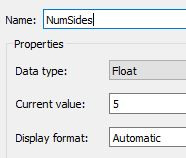D.2. VERTEX START AND END ANGLES

The angle between two consecutive vertices is given by 2*Pi/NumSides. If each vertex has an index assigned to it (starting from zero), then the AngleStart is obtained by multiplying its index by the angle between consecutive vertices. We will calculate the AngleStart for every occupation that lies within a side of the polygon. To determine the index we obtain the integer of the Angle divided by the angle between consecutive vertices. Similarly, an EndAngle can be obtained by adding 1 to the index. We express this as: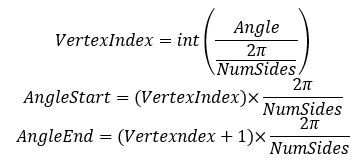The “+1e-9” term will be explained in the “D.4. LINE EQUATION FOR EACH SIDE” section below!

D.3. VERTEX START AND END COORDINATES

To obtain the XStart, XEnd, YStart, and YEnd of each vertex we use trigonometry.D.4. LINE EQUATION FOR EACH SIDE

A linear equation is obtained for each side of the polygon, using: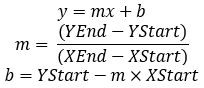Where m is the slope and b is the intercept.The slope is divided by XEnd-XStart. In some cases, a side of the polygon will be vertical to the X-axis, meaning that XEnd will be equal to XStart. This situation provides a division by 0 which causes problems in our calculations. Although there are several ways to address this, adding a small number (e.g. 1e-9) to AngleStart and AngleEnd mitigates the challenge by making XEnd and YEnd different from each other.

D.5. NUMBER OF BARS PER SIDE

To determine the number of occupations per side we divide the total number of occupations by the NumSides. We called this CF NumOccupPerSide.

D.6. INDEX OF THE VERTEX

We also require to know the index of the occupation at each vertex (XStartIndex). This is obtained by:D.7. X and Y COORDINATES

Here we obtain the X and Y coordinates where every occupation will be located. We will call these coordinates XPoly1 and YPoly1. For XPoly1 we want to determine all the x positions where each occupation will lie on:YPoly1 is calculated using the linear equation.

Finally, we separate the data based on the PathOrder. If the PathOrder is 0 we use the values of XPoly1 and YPoly1 previously calculated. However, if the PathOrder is 1, we need to add the NormGap. Given that the bars are perpendicular to the side, we use as an angle the average of the AngleStart and AngleEnd giving the following equation:E. ROTATING THE CHART

We may also want the ability to rotate the chart around the origin. To do this we use a rotation matrix. The equations are given by:We then create a parameter for the rotation angle (RotAngle) that can have a value between -180 and +180 degrees.F. VISUALIZING THE CHART

To visualize the chart we must make sure we are not aggregating the measures. Then we need to:

• Drag Gap to filter and remove Null values.

• Drag Occupation to the details.

• Drag the XCircle and YCircle for the circular chart, or XRot and YRot for the polygon chart, to the Columns and Rows. Compute Using Occupation!

• Change the Mark to Line.

• Drag AngleStart to Color.

Below we observe several examples of various polygons.We can also modify the angle of rotation of the polygon.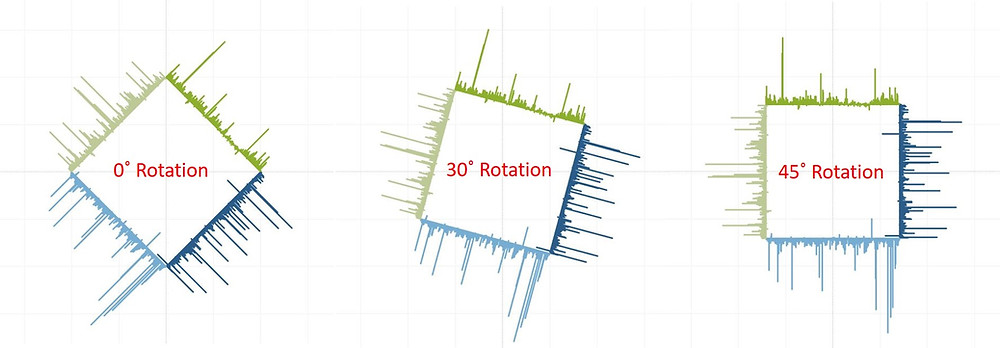Feel free to play with the dashboard below!

* There has been some controversy about the quality of the data for the analysis. For this post, we are focused on the chart design, and not on the accuracy of the data.